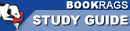Study Guide

Zero: The Biography of a Dangerous Idea Setting & Symbolism

This Study Guide consists of approximately 25 pages of chapter summaries, quotes, character analysis, themes, and more - everything you need to sharpen your knowledge of Zero.
 This section contains 234 words (approx. 1 page at 400 words per page) View a FREE sampleInfinity

This is Zero's constant companion and fellow troublemaker in the book.

Geometry

This is the science of shapes, size and space. Ancient ways of understanding geometry made zero hard to represent.

Algebra

This is the branch of mathematics concerned with relations and operations that zero made possible.

Classical Physics

This is the science invented by Isaac Newton for which zero made difficulties.

Calculus

This is the branch of math focused on limits and infinities that was invented by Leibniz and Newton. It was both made possible by zero and challenged by it.

Quantum Mechanics

This is the modern theory of the physics of the very small which was made possible by zero.

Thermodynamics

This is the science of heat made possible by zero.

General Relativity

This is the theory of very large physical structures invented by Einstein in which the zeroes involved with black holes are a...

 This section contains 234 words (approx. 1 page at 400 words per page) View a FREE sample